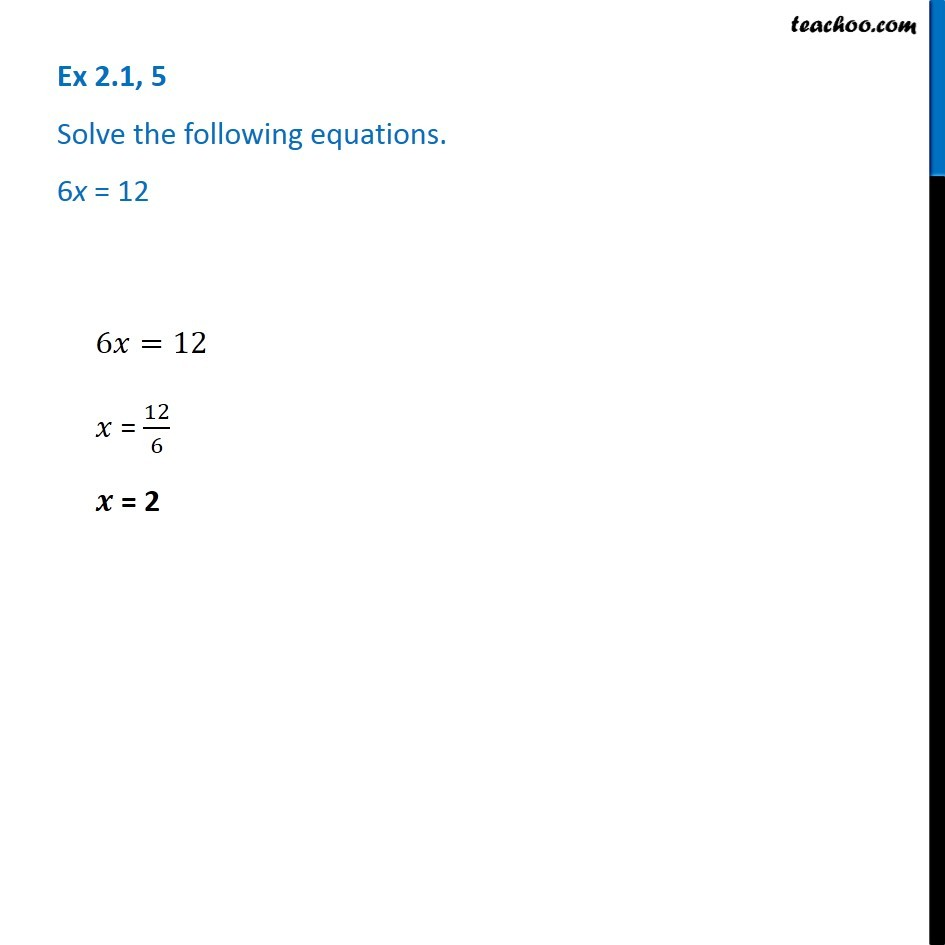Solving easy equations

Chapter 2 Class 8 Linear Equations in One Variable
Serial order wiseLearn in your speed, with individual attention - Teachoo Maths 1-on-1 Class

### Transcript

Question 5 - Chapter 2 Class 8 - Linear Equations in one variable - NCERT Book Solve the following equations. 6x = 12 6x = 12 Taking 6 to the right side x = 12/6 x = 2